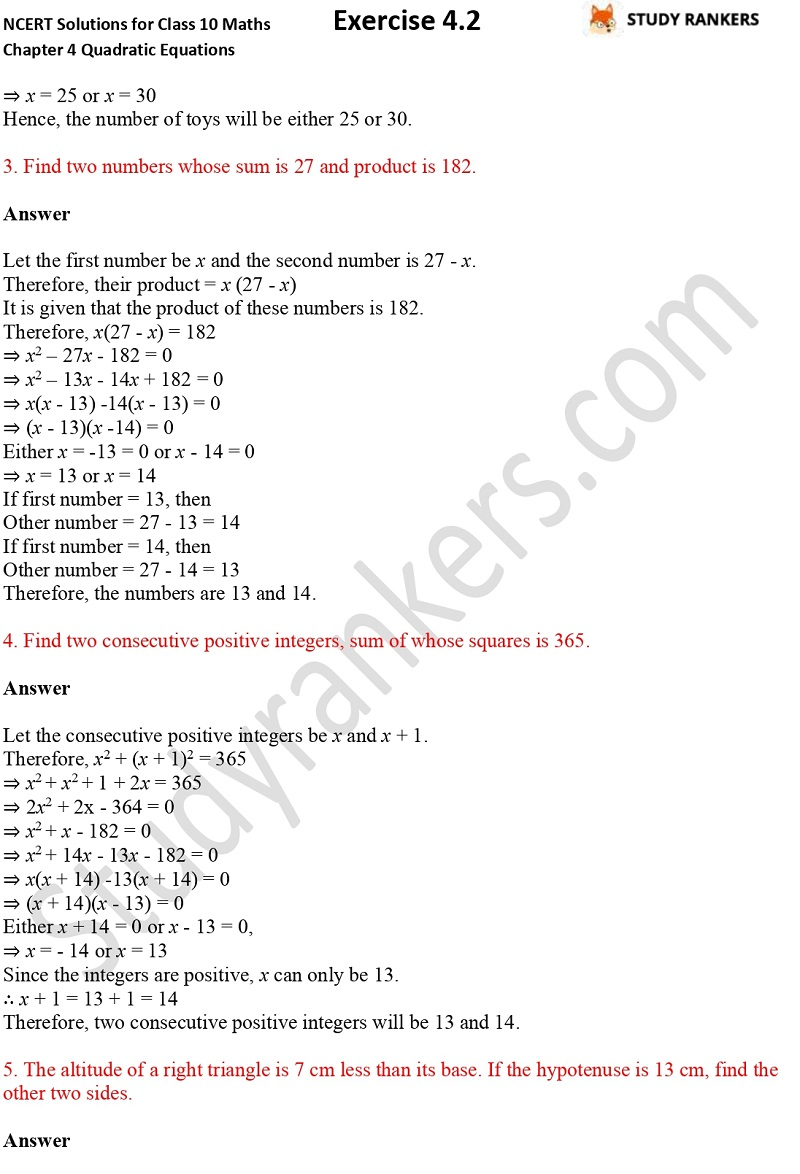## NCERT Solutions for Class 10 Maths Chapter 4 Quadratic Equations Exercise 4.2

If you're need of NCERT Solutions for Class 10 Maths the you're at right place. Here, you will find Chapter 4 Quadratic Equations Exercise 4.2 NCERT Solutions for Class 10 Maths that will help you in scoring more marks in the examination. These will prove useful in completing your homework on time and solve difficult problems.

There are total six questions in whole exercise. In the first, you have to find the roots of the given quadratic equations by factorisation while others are word problems.